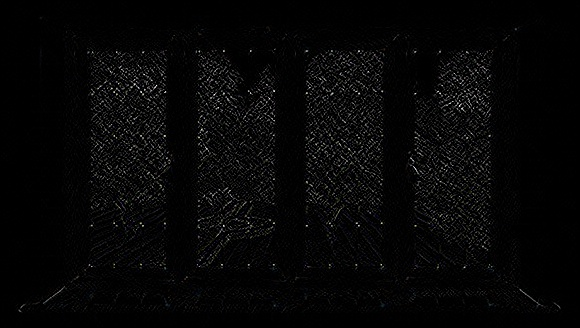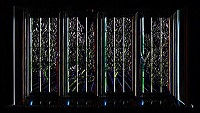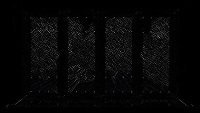# OpenCV - Sobel Operator

Using the sobel operation, you can detect the edges of an image in both horizontal and vertical directions. You can apply sobel operation on an image using the method sobel(). Following is the syntax of this method −

```Sobel(src, dst, ddepth, dx, dy)
```

This method accepts the following parameters −

• src − An object of the class Mat representing the source (input) image.

• dst − An object of the class Mat representing the destination (output) image.

• ddepth − An integer variable representing the depth of the image (-1)

• dx − An integer variable representing the x-derivative. (0 or 1)

• dy − An integer variable representing the y-derivative. (0 or 1)

### Example

The following program demonstrates how to perform Sobel operation on a given image.

```import org.opencv.core.Core;
import org.opencv.core.Mat;

import org.opencv.imgcodecs.Imgcodecs;
import org.opencv.imgproc.Imgproc;

public class SobelTest {
public static void main(String args[]) {

// Reading the Image from the file and storing it in to a Matrix object
String file ="E:/OpenCV/chap16/sobel_input.jpg";

// Creating an empty matrix to store the result
Mat dst = new Mat();

// Applying sobel on the Image
Imgproc.Sobel(src, dst, -1, 1, 1);

// Writing the image
Imgcodecs.imwrite("E:/OpenCV/chap16/sobel_output.jpg", dst);

System.out.println("Image processed");
}
}
```

Assume that following is the input image sobel_input.jpg specified in the above program.### Output

On executing the program, you will get the following output −

```Image Processed
```

If you open the specified path, you can observe the output image as follows −## sobel Variants

On passing different values to the last to parameters (dx and dy) (among 0 and 1), you will get different outputs −

```// Applying sobel on the Image
Imgproc.Sobel(src, dst, -1, 1, 1);
```

The following table lists various values for the variables dx and dy of the method Sobel() and their respective outputs.

X-derivative Y-derivative Output
0 11 01 1